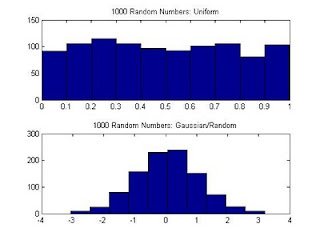## Monday, August 9, 2010

### Creating Random Numbers in MatlabGenerating a matrix of random numbers:
The following code generates a 1 by 1000 matrix of 1000 random numbers between 0 and 1.

rand(1,1000);

Generating a Number between a and b
:
The following code generates a uniform whole random number between 1 (a) and 100 (b).

a = 1;
b = 100;
x = round(a + (b-a) * rand);

a and b can be adjusted as desired. For a normal Gaussian random number, the randn function can be used in place of rand above.

Here is the Matlab code for the plot above.

%% Generating random numbers in Matlab
% James Eastham
% Member, IEEE
% Revision: R1-0-0
clear all;
close all;
%% Generating 1000 random numbers using rand and randn
% This section generates a matrix of 1000
% random numbers between 0 and 1 using the
% rand and randn functions. The rand function
% creates a uniform distribution while the
% randn function generates a normal (Gaussian)
% distribution
x = rand(1,1000); %generates a 1 by 1000 matrix
figure('Color',[1 1 1]);
subplot(2,1,1);
hist(x);
title('1000 Random Numbers: Uniform');
x = randn(1,1000); %generates a 1 by 1000 matrix
subplot(2,1,2);
hist(x);
title('1000 Random Numbers: Gaussian/Random');
%% Generating a random number between 1 and 100
% The following code demonstrates a method
% for generating a random number between
% 1 (a) and 100 (b). The round function is
% used to round the number to the closest whole
% number.
a = 1;
b = 100;
x = round(a + (b-a) * rand)

#### 2 comments:

Phlee86 said...

how does this generate random numbers from 1 to 100 if your plot shows negative numbers?

RFWarrior said...

The plot shows 1000 random numbers using the randn function. You can use the help function in Matlab to see how this work.

The second block of code generates a random number from 1 to 100.

-James